Publicité

# Capitulo 3 del libro TERMODINAMICA

13 Nov 2012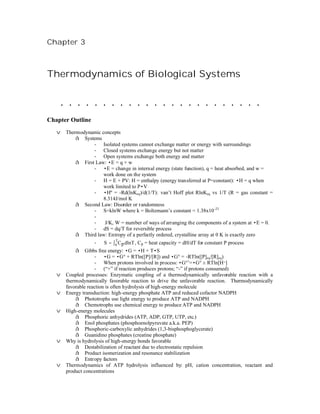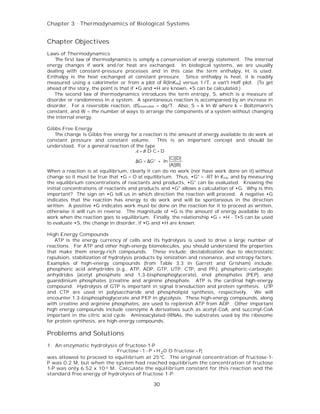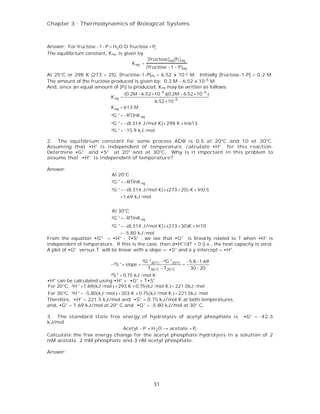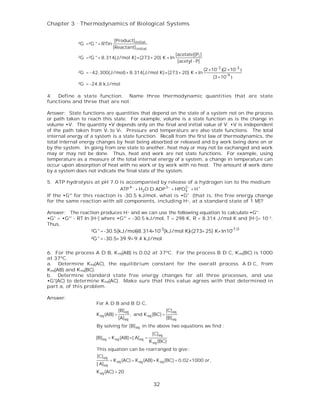Publicité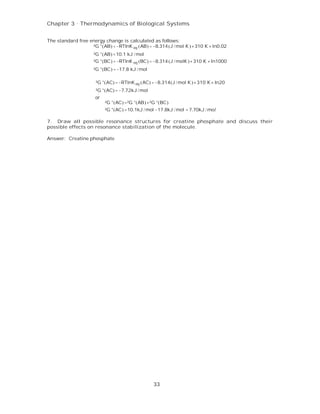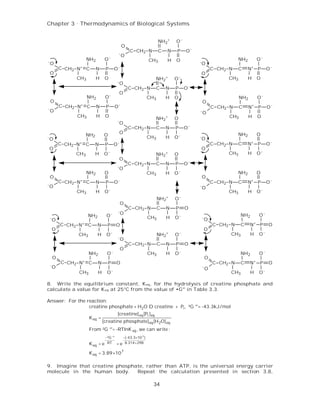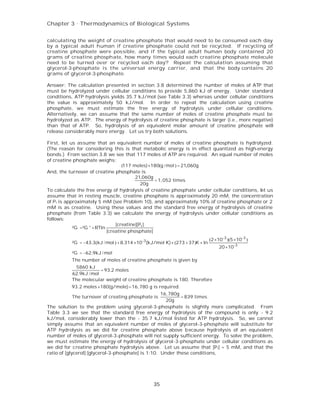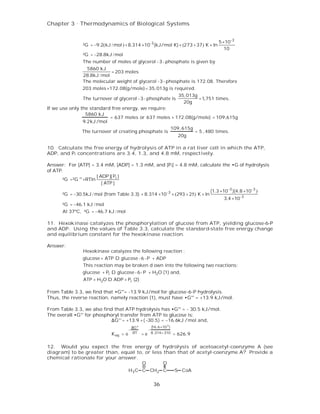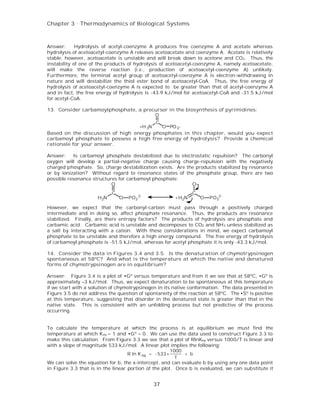Publicité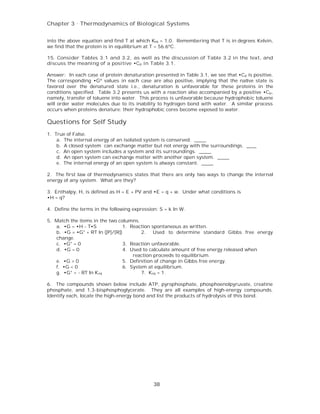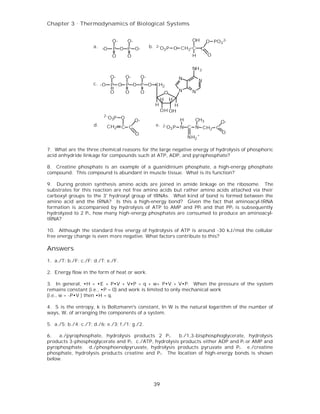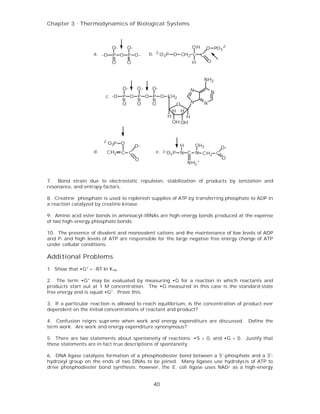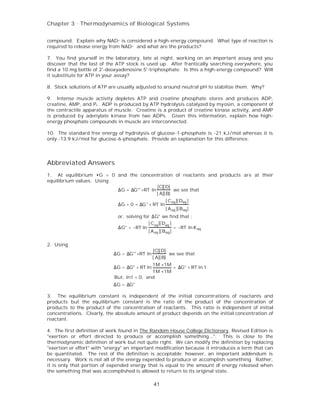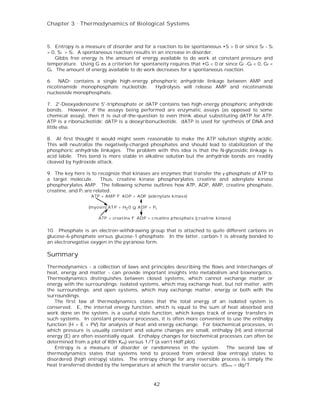Publicité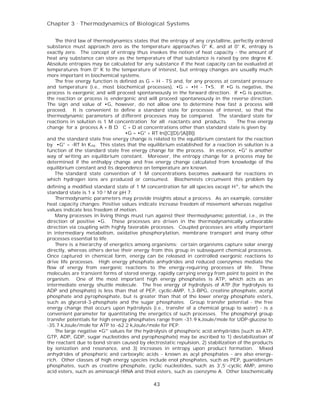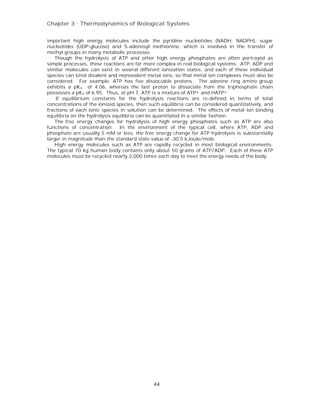Prochain SlideShareScreening models of anti psychotic drugs-converted
Chargement dans ... 3
1 sur 16
Publicité

### Capitulo 3 del libro TERMODINAMICA

1. Chapter 3 Thermodynamics of Biological Systems ........................ Chapter Outline v Thermodynamic concepts å Systems - Isolated systems cannot exchange matter or energy with surroundings - Closed systems exchange energy but not matter - Open systems exchange both energy and matter å First Law: •E = q + w - •E = change in internal energy (state function), q = heat absorbed, and w = work done on the system - H = E + PV: H = enthalpy (energy transferred at P=constant): •H = q when work limited to P•V - •Hº = -Rd(lnKeq)/d(1/T): van’t Hoff plot RlnKeq vs 1/T (R = gas constant = 8.314J/mol K å Second Law: Disorder or randomness - S=klnW where k = Boltzmann’s constant = 1.38x10 -23 - - J/K, W = number of ways of arranging the components of a system at •E = 0. - dS = dq/T for reversible process å Third law: Entropy of a perfectly ordered, crystalline array at 0 K is exactly zero - S = ∫ 0 C p dlnT , Cp = heat capacity = dH/dT for constant P process T å Gibbs free energy: •G = •H + T•S - •G = •G° + RTln([P]/[R]) and •G° = -RTln([P]eq/[R]eq) - When protons involved in process: •G°’=•G° ± RTln[H+] - (“+” if reaction produces protons; “-” if protons consumed) v Coupled processes: Enzymatic coupling of a thermodynamically unfavorable reaction with a thermodynamically favorable reaction to drive the unfavorable reaction. Thermodynamically favorable reaction is often hydrolysis of high-energy molecule v Energy transduction: high-energy phosphate ATP and reduced cofactor NADPH å Phototrophs use light energy to produce ATP and NADPH å Chemotrophs use chemical energy to produce ATP and NADPH v High-energy molecules å Phosphoric anhydrides (ATP, ADP, GTP, UTP, etc.) å Enol phosphates (phosphoenolpyruvate a.k.a. PEP) å Phosphoric-carboxylic anhydrides (1,3-bisphosphoglycerate) å Guanidino phosphates (creatine phosphate) v Why is hydrolysis of high-energy bonds favorable å Destabilization of reactant due to electrostatic repulsion å Product isomerization and resonance stabilization å Entropy factors v Thermodynamics of ATP hydrolysis influenced by: pH, cation concentration, reactant and product concentrations
2. Chapter 3 . Thermodynamics of Biological Systems Chapter Objectives Laws of Thermodynamics The first law of thermodynamics is simply a conservation of energy statement. The internal energy changes if work and/or heat are exchanged. In biological systems, we are usually dealing with constant-pressure processes and in this case the term enthalpy, H, is used. Enthalpy is the heat exchanged at constant pressure. Since enthalpy is heat, it is readily measured using a calorimeter or from a plot of R(lnKeq) versus 1/T, a van't Hoff plot. (To get ahead of the story, the point is that if •G and •H are known, •S can be calculated.) The second law of thermodynamics introduces the term entropy, S, which is a measure of disorder or randomness in a system. A spontaneous reaction is accompanied by an increase in disorder. For a reversible reaction, dSreversible = dq/T. Also, S = k ln W where k = Boltzmann's constant, and W = the number of ways to arrange the components of a system without changing the internal energy. Gibbs Free Energy The change is Gibbs free energy for a reaction is the amount of energy available to do work at constant pressure and constant volume. This is an important concept and should be understood. For a general reaction of the type A + B D C+ D [C][D] ∆G = ∆G° + ln [A][B] When a reaction is at equilibrium, clearly it can do no work (nor have work done on it) without change so it must be true that •G = O at equilibrium. Thus, •G° = -RT ln K eq, and by measuring the equilibrium concentrations of reactants and products, •G° can be evaluated. Knowing the initial concentrations of reactants and products and •G° allows a calculation of •G. Why is this important? The sign on •G tell us in which direction the reaction will proceed. A negative •G indicates that the reaction has energy to do work and will be spontaneous in the direction written. A positive •G indicates work must be done on the reaction for it to proceed as written, otherwise it will run in reverse. The magnitude of •G is the amount of energy available to do work when the reaction goes to equilibrium. Finally, the relationship •G = •H - T•S can be used to evaluate •S, the change in disorder, if •G and •H are known. High Energy Compounds ATP is the energy currency of cells and its hydrolysis is used to drive a large number of reactions. For ATP and other high-energy biomolecules, you should understand the properties that make them energy-rich compounds. These include: destabilization due to electrostatic repulsion, stabilization of hydrolysis products by ionization and resonance, and entropy factors. Examples of high-energy compounds (from Table 3.3 in Garrett and Grisham) include: phosphoric acid anhydrides (e.g., ATP, ADP, GTP, UTP, CTP, and PP i), phosphoric-carboxylic anhydrides (acetyl phosphate and 1,3-bisphosphoglycerate), enol phosphates (PEP), and guanidinium phosphates (creatine and arginine phosphate. ATP is the cardinal high-energy compound. Hydrolysis of GTP is important in signal transduction and protein synthesis. UTP and CTP are used in polysaccharide and phospholipid synthesis, respectively. We will encounter 1,3-bisphosphoglycerate and PEP in glycolysis. These high-energy compounds, along with creatine and arginine phosphates, are used to replenish ATP from ADP. Other important high energy compounds include coenzyme A derivatives such as acetyl-CoA, and succinyl-CoA important in the citric acid cycle. Aminoacylated-tRNAs, the substrates used by the ribosome for protein synthesis, are high-energy compounds. Problems and Solutions 1. An enzymatic hydrolysis of fructose-1-P Fructose −1 − P + H 2 O D fructose + Pi was allowed to proceed to equilibrium at 25°C. The original concentration of fructose-1- P was 0.2 M, but when the system had reached equilibrium the concentration of fructose 1-P was only 6.52 x 10 -5 M. Calculate the equilibrium constant for this reaction and the standard free energy of hydrolysis of fructose 1-P. 30
3. Chapter 3 . Thermodynamics of Biological Systems Answer: For fructose −1− P + H2O D fructose + Pi The equilibrium constant, Keq, is given by [fructose]eq [Pi ] eq K eq = [ fructose −1 − P]eq At 25°C or 298 K (273 + 25), [fructose-1-P]eq = 6.52 x 10 -5 M. Initially [fructose-1-P] = 0.2 M. The amount of the fructose produced is given by: 0.2 M - 6.52 x 10 -5 M. And, since an equal amount of [Pi] is produced, Keq may be written as follows: (0.2M − 6.52 ×10 −5 )(0.2M − 6.52 ×10−5 ) K eq = 6.52 ×10−5 K eq = 613 M ²G ° = −RTlnK eq ²G ° = −(8.314 J/mol K)× 298 K × ln613 ²G ° = −15.9 kJ / mol 2. The equilibrium constant for some process ADB is 0.5 at 20°C and 10 at 30°C. Assuming that •H° is independent of temperature, calculate •H° for this reaction. Determine •G° and •S° at 20° and at 30°C. Why is it important in this problem to assume that •H° is independent of temperature? Answer: At 20°C ²G ° = −RTlnK eq ²G ° = −(8.314 J/mol K)× (273 + 20) K × ln0.5 =1.69 kJ / mol At 30°C ²G ° = −RTlnK eq ²G ° = −(8.314 J/mol K)× (273 + 30)K × ln10 = −5.80 kJ / mol From the equation •G° = •H° - T•S° , we see that •G° is linearly related to T when •H° is independent of temperature. If this is the case, then d•H°/dT = 0 (i.e., the heat capacity is zero). A plot of •G° versus T will be linear with a slope =- •S° and a y intercept = •H°. ²G °30°C −²G °20°C −5.8 −1.69 −²S ° = slope = = T30°C − T20°C 30 − 20 ²S ° = 0.75 kJ / mol K •H° can be calculated using •H° = •G° + T•S°. For 20°C, ²H ° =1.69(kJ / mol ) + 293 K × 0.75(kJ / mol K ) = 221.5kJ / mol For 30°C, ²H ° = −5.80(kJ / mol ) + 303 K × 0.75(kJ / mol K ) = 221.5kJ / mol Therefore, •H° = 221.5 kJ/mol and •S° = 0.75 kJ/mol K at both temperatures, and, •G° = 1.69 kJ/mol at 20° C and •G° = -5.80 kJ/mol at 30° C. 3. The standard state free energy of hydrolysis of acetyl phosphate is •G° = -42.3 kJ/mol. Acetyl − P + H 2O → acetate + Pi Calculate the free energy change for the acetyl phosphate hydrolysis in a solution of 2 mM acetate, 2 mM phosphate and 3 nM acetyl phosphate. Answer: 31
4. Chapter 3 . Thermodynamics of Biological Systems [Product] initial ²G =²G ° + RTln [Reactant] initial [acetate][Pi ] ²G =²G ° + 8.314(J/mol K)×(273+ 20) K × ln [acetyl - P] (2 ×10-3 )(2 ×10-3 ) ²G = −42,300(J/mol)+ 8.314(J/mol K)×(273+ 20) K × ln (3 ×10-9 ) ²G = -24.8 kJ/mol 4. Define a state function. Name three thermodynamic quantities that are state functions and three that are not. Answer: State functions are quantities that depend on the state of a system not on the process or path taken to reach this state. For example, volume is a state function as is the change in volume •V. The quantity •V depends only on the final and initial value of V; •V is independent of the path taken from Vi to V f. Pressure and temperature are also state functions. The total internal energy of a system is a state function. Recall from the first law of thermodynamics, the total internal energy changes by heat being absorbed or released and b y work being done on or by the system. In going from one state to another, heat may or may not be exchanged and work may or may not be done. Thus, heat and work are not state functions. For example, using temperature as a measure of the total internal energy of a system, a change in temperature can occur upon absorption of heat with no work or by work with no heat. The amount of work done by a system does not indicate the final state of the system. 5. ATP hydrolysis at pH 7.0 is accompanied by release of a hydrogen ion to the medium ATP 4− + H2O D ADP 3− + HPO2− + H + 4 If the •G°' for this reaction is -30.5 kJ/mol, what is •G° (that is, the free energy change for the same reaction with all components, including H+, at a standard state of 1 M)? Answer: The reaction produces H + and we can use the following equation to calculate •G°: •G° = •G°' - RT ln [H +] where •G°' = -30.5 kJ/mol, T = 298 K, R = 8.314 J/mol K and [H +]= 10 -7. Thus, -8.314×10-3(kJ/mol K) (273+ 25) K× ln10-7.0 ²G ° = -30.5(kJ/mol) × ²G ° = -30.5+ 39.9= 9.4 kJ/mol 6. For the process A D B, K eq(AB) is 0.02 at 37ºC. For the process B D C, K eq(BC) is 1000 at 37ºC. a. Determine Keq(AC), the equilibrium constant for the overall process A D C, from Keq(AB) and K eq(BC). b. Determine standard state free energy changes for all three processes, and use •G°(AC) to determine Keq(AC). Make sure that this value agrees with that determined in part a, of this problem. Answer: For A D B and B D C, [B] eq [C] eq K eq (AB) = , and K eq (BC) = [A] eq [B] eq By solving for [B] eq in the above two equations we find : [C] eq [B] eq = K eq (AB) ×[ A] eq = K eq (BC) This equation can be rearranged to give : [C] eq = K eq (AC) = K eq (AB) × K eq (BC) = 0.02 ×1000 or, [ A] eq K eq (AC) = 20 32
5. Chapter 3 . Thermodynamics of Biological Systems The standard free energy change is calculated as follows: ²G °(AB) = −RTlnK eq (AB) = −8.314 (J / mol K ) × 310 K × ln0.02 ²G °(AB) =10.1 kJ / mol ²G °(BC) = −RTlnK eq (BC) = −8.314 (J / molK ) × 310 K × ln1000 ²G °(BC) = −17.8 kJ / mol ²G °(AC) = −RTlnK eq (AC) = −8.314(J / mol K ) × 310 K × ln20 ²G °(AC) = −7.72kJ / mol or ²G °(AC) =²G °(AB) +²G °(BC) ²G °(AC) =10.1kJ / mol −17.8kJ / mol = 7.70kJ / mol 7. Draw all possible resonance structures for creatine phosphate and discuss their possible effects on resonance stabilization of the molecule. Answer: Creatine phosphate 33
6. Chapter 3 . Thermodynamics of Biological Systems NH2+ O- O C CH2 N C N P O- - O NH2 O- CH3 H O NH2 O- - - O O C CH2 N+ C N P O- C CH2 N C N+ P O- O O CH3 H O NH2+ O- CH3 H O - O C CH2 N C N P O- O NH2 O- CH3 H O NH2 O- O O C CH2 N+ C N P O- C CH2 N C N+ P O- - O - O CH3 H O CH3 H O NH2+ O - O C CH2 N C N P O- O NH2 O -O NH2 O CH3 H O- -O C CH2 N+ C N P O- C CH2 N C N+ P O- O O CH3 H O- NH2+ O CH3 H O- O C CH2 N C N P O- -O NH2 O CH3 H O- NH2 O O O C CH2 N+ C N P O- C CH2 N C N+ P O- -O -O CH3 H O- CH3 H O- NH2+ O- O C CH2 N C N P O -O NH2 O- NH2 O- -O CH3 H O- -O C CH2 N+ C N P O C CH2 N C N+ P O O O CH3 H O- NH2+ O- CH3 H O- -O C CH2 N C N P O O NH2 O- CH3 H O- NH2 O- O O C CH2 N+ C N P O C CH2 N C N+ P O -O -O CH3 H O- CH3 H O- 8. Write the equilibrium constant, Keq, for the hydrolysis of creatine phosphate and calculate a value for K eq at 25°C from the value of •G°' in Table 3.3. Answer: For the reaction: creatine phosphate + H2O D creatine + Pi , ²G °'= -43.3kJ/mol [creatine] eq [Pi ]eq K eq = [creatine phosphate] eq [H 2O] eq From ²G °'= -RTlnK eq , we can write : −²G °' −(-43.3×10 3 ) K eq = e RT = e 8.314×298 K eq = 3.89×10 7 9. Imagine that creatine phosphate, rather than ATP, is the universal energy carrier molecule in the human body. Repeat the calculation presented in section 3.8, 34
7. Chapter 3 . Thermodynamics of Biological Systems calculating the weight of creatine phosphate that would need to be consumed each day by a typical adult human if creatine phosphate could not be recycled. If recycling of creatine phosphate were possible, and if the typical adult human body contained 20 grams of creatine phosphate, how many times would each creatine phosphate molecule need to be turned over or recycled each day? Repeat the calculation assuming that glycerol-3-phosphate is the universal energy carrier, and that the body contains 20 grams of glycerol-3-phosphate. Answer: The calculation presented in section 3.8 determined the number of moles of ATP that must be hydrolyzed under cellular conditions to provide 5,860 kJ of energy. Under standard conditions, ATP hydrolysis yields 35.7 kJ/mol (see Table 3.3) whereas under cellular conditions the value is approximately 50 kJ/mol. In order to repeat the calculation using creatine phosphate, we must estimate the free energy of hydrolysis under cellular conditions. Alternatively, we can assume that the same number of moles of creatine phosphate must be hydrolyzed as ATP. The energy of hydrolysis of creatine phosphate is larger (i.e., more negative) than that of ATP. So, hydrolysis of an equivalent molar amount of creatine phosphate will release considerably more energy. Let us try both solutions. First, let us assume that an equivalent number of moles of creatine phosphate is hydrolyzed. (The reason for considering this is that metabolic energy is in effect quantized as high-energy bonds.) From section 3.8 we see that 117 moles of ATP are required. An equal number of moles of creatine phosphate weighs: (117 moles) ×180(g / mol ) = 21,060g And, the turnover of creatine phosphate is 21,060g =1,052 times 20g To calculate the free energy of hydrolysis of creatine phosphate under cellular conditions, let us assume that in resting muscle, creatine phosphate is approximately 20 mM, the concentration of P i is approximately 5 mM (see Problem 10), and approximately 10% of creatine phosphate or 2 mM is as creatine. Using these values and the standard free energy of hydrolysis of creatine phosphate (from Table 3.3) we calculate the energy of hydrolysis under cellular conditions as follows: [creatine][Pi ] ²G =²G ° + RTln [creatine phosphate] (2 ×10-3 )(5 ×10-3 ) ²G = −43.3(kJ / mol ) + 8.314 ×10 -3 (kJ/mol K) × (273 + 37)K × ln 20 ×10-3 ²G = −62.9kJ / mol The number of moles of creatine phosphate is given by 5860 kJ = 93.2 moles 62.9kJ / mol The molecular weight of creatine phosphate is 180. Therefore 93.2 moles ×180(g/mole) =16,780 g is required. 16,780g The turnover of creating phosphate is = 839 times. 20g The solution to the problem using glycerol-3-phosphate is slightly more complicated. From Table 3.3 we see that the standard free energy of hydrolysis of the compound is only - 9.2 kJ/mol, considerably lower than the - 35.7 kJ/mol listed for ATP hydrolysis. So, we cannot simply assume that an equivalent number of moles of glycerol-3-phosphate will substitute for ATP hydrolysis as we did for creatine phosphate above b ecause hydrolysis of an equivalent number of moles of glycerol-3-phosphate will not supply sufficient energy. To solve the problem, we must estimate the energy of hydrolysis of glycerol-3-phosphate under cellular conditions as we did for creatine phosphate hydrolysis above. Let us assume that [P i] = 5 mM, and that the ratio of [glycerol]:[glycerol-3-phosphate] is 1:10. Under these conditions, 35
8. Chapter 3 . Thermodynamics of Biological Systems 5 ×10-3 ²G = −9.2(kJ / mol ) + 8.314 ×10-3 (kJ/mol K) × (273 + 37) K × ln 10 ²G = −28.8kJ / mol The number of moles of glycerol - 3 - phosphate is given by 5860 kJ = 203 moles 28.8kJ / mol The molecular weight of glycerol -3 - phosphate is 172.08. Therefore 203 moles ×172.08(g/mole)= 35,013g is required. 35,013g The turnover of glycerol - 3 - phosphate is =1,751 times. 20g If we use only the standard free energy, we require: 5860 kJ = 637 moles or 637 moles × 172.08(g/mole) = 109,615g 9.2kJ /mol 109, 615g The turnover of creating phosphate is = 5 , 480 times. 20g 10. Calculate the free energy of hydrolysis of ATP in a rat liver cell in which the ATP, ADP, and Pi concentrations are 3.4, 1.3, and 4.8 mM, respectively. Answer: For [ATP] = 3.4 mM, [ADP] = 1.3 mM, and [Pi] = 4.8 mM, calculate the •G of hydrolysis of ATP. [ ADP ][ Pi ] ²G =²G °' +RTln [ ATP ] (1.3 ×10 -3 )(4.8 ×10 -3 ) ²G = −30.5kJ / mol (from Table 3.3) + 8.314 ×10 -3 × (293 + 25) K × ln 3.4 ×10 -3 ²G = −46.1 kJ / mol At 37ºC, ²G = −46.7 kJ / mol 11. Hexokinase catalyzes the phosphorylation of glucose from ATP, yielding glucose-6-P and ADP. Using the values of Table 3.3, calculate the standard-state free energy change and equilibrium constant for the hexokinase reaction. Answer: Hexokinase catalyzes the following reaction : glucose + ATP D glucose -6 -P + ADP This reaction may be broken d own into the following two reactions: glucose + Pi D glucose - 6 - P + H2O (1) and, ATP + H2O D ADP + Pi (2) From Table 3.3, we find that •G°'= -13.9 kJ/mol for glucose-6-P hydrolysis. Thus, the reverse reaction, namely reaction (1), must have •G°' = +13.9 kJ/mol. From Table 3.3, we also find that ATP hydrolysis has •G°' = - 30.5 kJ/mol. The overall •G°' for phosphoryl transfer from ATP to glucose is: ∆G°' = +13.9 + ( −30 .5) = −16.6kJ /mol and, ∆G°' (16.6 ×10 3 ) − − K eq = e RT =e 8.314× 310 = 626.9 12. Would you expect the free energy of hydrolysis of acetoacetyl-coenzyme A (see diagram) to be greater than, equal to, or less than that of acetyl-coenzyme A? Provide a chemical rationale for your answer. O O H3 C C CH2 C S CoA 36
9. Chapter 3 . Thermodynamics of Biological Systems Answer: Hydrolysis of acetyl-coenzyme A produces free coenzyme A and acetate whereas hydrolysis of acetoacetyl-coenzyme A releases acetoacetate and coenzyme A. Acetate is relatively stable; however, acetoacetate is unstable and will break down to acetone and CO2. Thus, the instability of one of the products of hydrolysis of acetoacetyl-coenzyme A, namely acetoacetate, will make the reverse reaction (i.e., production of acetoacetyl-coenzyme A) unlikely. Furthermore, the terminal acetyl group of acetoacetyl-coenzyme A is electron-withdrawing in nature and will destabilize the thiol ester bond of acetoacetyl-CoA. Thus, the free energy of hydrolysis of acetoacetyl-coenzyme A is expected to be greater than that of acetyl-coenzyme A and in fact, the free energy of hydrolysis is -43.9 kJ/mol for acetoacetyl-CoA and -31.5 kJ/mol for acetyl-CoA. 13. Consider carbamoylphosphate, a precursor in the biosynthesis of pyrimidines: O C +H 3N O PO 3- Based on the discussion of high energy phosphates in this chapter, would you expect carbamoyl phosphate to possess a high free energy of hydrolysis? Provide a chemical rationale for your answer. Answer: Is carbamoyl phosphate destabilized due to electrostatic repulsion? The carbonyl oxygen will develop a partial-negative charge causing charge-repulsion with the negatively charged phosphate. So, charge destabilization exists. Are the products stabilized by resonance or by ionization? Without regard to resonance states of the phosphate group, there are two possible resonance structures for carbamoyl phosphate: O O- C C H2N O PO3 2- +H2N O PO32- However, we expect that the carbonyl-carbon must pass through a positively charged intermediate and in doing so, affect phosphate resonance. Thus, the products are resonance stabilized. Finally, are there entropy factors? The products of hydrolysis are phosphate and carbamic acid. Carbamic acid is unstable and decomposes to CO2 and NH 3 unless stabilized as a salt by interacting with a cation. With these considerations in mind, we expect carbamoyl phosphate to be unstable and therefore a high energy compound. The free energy of hydrolysis of carbamoyl phosphate is -51.5 kJ/mol, whereas for acetyl phosphate it is only -43.3 kJ/mol. 14. Consider the data in Figures 3.4 and 3.5. Is the denaturation of chymotrypsinogen spontaneous at 58ºC? And what is th e temperature at which the native and denatured forms of chymotrypsinogen are in equilibrium? Answer: Figure 3.4 is a plot of •Gº versus temperature and from it we see that at 58ºC, •Gº is approximately –3 kJ/mol. Thus, we expect denaturation to be spontaneous at this temperature if we start with a solution of chymotrypsinogen in its native conformation. The data presented in Figure 3.5 do not address the question of spontaneity of the reaction at 58ºC. The •Sº is positive at this temperature, suggesting that disorder in the denatured state is greater than that in the native state. This is consistent with an unfolding process but not predictive of the process occurring. To calculate the temperature at which the process is at equilibrium we must find the temperature at which Keq = 1 and •Gº = 0. We can use the data used to construct Figure 3.3 to make this calculation. From Figure 3.3 we see that a plot of RlnKeq versus 1000/T is linear and with a slope of magnitude 533 kJ/mol. A linear plot implies the following: 1000 R ln K eq = - 533 × + b T We can solve the equation for b, the x-intercept, and can evaluate b by using any one data point in Figure 3.3 that is in the linear portion of the plot. Once b is evaluated, we can substitute it 37
10. Chapter 3 . Thermodynamics of Biological Systems into the above equation and find T at which Keq = 1.0. Remembering that T is in degrees Kelvin, we find that the protein is in equilibrium at T = 56.6ºC. 15. Consider Tables 3.1 and 3.2, as well as the discussion of Table 3.2 in the text, and discuss the meaning of a positive •C p in Table 3.1. Answer: In each case of protein denaturation presented in Table 3.1, we see that •Cp is positive. The corresponding •Gº values in each case are also positive, implying that the native state is favored over the denatured state i.e., denaturation is unfavorable for these proteins in the conditions specified. Table 3.2 presents us with a reaction also accompanied by a positive •Cp, namely, transfer of toluene into water. This process is unfavorable because hydrophobic toluene will order water molecules due to its inability to hydrogen bond with water. A similar process occurs when proteins denature: their hydrophobic cores become exposed to water. Questions for Self Study 1. True of False. a. The internal energy of an isolated system is conserved. _____ b. A closed system can exchange matter but not energy with the surroundings. ____ c. An open system includes a system and its surroundings. _____ d. An open system can exchange matter with another open system. _____ e. The internal energy of an open system is always constant. _____ 2. The first law of thermodynamics states that there are only two ways to change the internal energy of any system. What are they? 3. Enthalpy, H, is defined as H = E + PV and •E = q + w. Under what conditions is •H = q? 4. Define the terms in the following expression: S = k ln W. 5. Match the items in the two columns. a. •G = •H - T•S 1. Reaction spontaneous as written. b. •G = •G° + RT ln ([P]/[R]) 2. Used to determine standard Gibbs free energy change. c. •G° = 0 3. Reaction unfavorable. d. •G = 0 4. Used to calculate amount of free energy released when reaction proceeds to equilibrium. e. •G > 0 5. Definition of change in Gibbs free energy. f. •G < 0 6. System at equilibrium. g. •G° = - RT ln K eq 7. Keq = 1. 6. The compounds shown below include ATP, pyrophosphate, phosphoenolpyruvate, creatine phosphate, and 1,3-bisphosphoglycerate. They are all examples of high-energy compounds. Identify each, locate the high-energy bond and list the products of hydrolysis of this bond. 38
11. Chapter 3 . Thermodynamics of Biological Systems O- O- OH O PO3 2- a. -O P O P O- b. 2- O3 P O CH2 C C O O H O NH 2 O- O- O- N N c. -O P O P O P O CH2 O O O O N N H H H H OH OH 2- O3P O H CH3 O- O- d. CH2 C C e. 2- O P N C N CH2 C 3 O O NH2 + 7. What are the three chemical reasons for the large negative energy of hydrolysis of phosphoric acid anhydride linkage for compounds such at ATP, ADP, and pyrophosphate? 8. Creatine phosphate is an example of a guanidinium phosphate, a high-energy phosphate compound. This compound is abundant in muscle tissue. What is its function? 9. During protein synthesis amino acids are joined in amide linkage on the ribosome. The substrates for this reaction are not free amino acids but rather amino acids attached via their carboxyl groups to the 3' hydroxyl group of tRNAs. What kind of bond is formed between the amino acid and the tRNA? Is this a high-energy bond? Given the fact that aminoacyl-tRNA formation is accompanied by hydrolysis of ATP to AMP and PPi and that PP i is subsequently hydrolyzed to 2 P i, how many high-energy phosphates are consumed to produce an aminoacyl- tRNA? 10. Although the standard free energy of hydrolysis of ATP is around -30 kJ/mol the cellular free energy change is even more negative. What factors contribute to this? Answers 1. a./T; b./F; c./F; d./T; e./F. 2. Energy flow in the form of heat or work. 3. In general, •H = •E + P•V + V•P = q + w+ P•V + V•P. When the pressure of the system remains constant (i.e., •P = 0) and work is limited to only mechanical work (i.e., w = -P•V ) then •H = q. 4. S is the entropy, k is Boltzmann's constant, ln W is the natural logarithm of the number of ways, W, of arranging the components of a system. 5. a./5; b./4; c./7; d./6; e./3; f./1; g./2. 6. a./pyrophosphate, hydrolysis products 2 P i. b./1,3-bisphosphoglycerate, hydrolysis products 3-phosphoglycerate and Pi. c./ATP, hydrolysis products either ADP and Pi or AMP and pyrophosphate. d./phosphoenolpyruvate, hydrolysis products pyruvate and P i. e./creatine phosphate, hydrolysis products creatine and P i. The location of high-energy bonds is shown below. 39
12. Chapter 3 . Thermodynamics of Biological Systems O- O- OH O PO3 2- a. -O b. 2- P O P O- O3 P O CH2 C C O O H O NH2 O- O- O- N N c. -O P O P O P O CH2 O O O O N N H H H H OH OH 2- O3P O H CH3 O- O- d. CH2 C C e. 2- O P 3 N C N CH2 C O O NH2 + 7. Bond strain due to electrostatic repulsion, stabilization of products by ionization and resonance, and entropy factors. 8. Creatine phosphate is used to replenish supplies of ATP by transferring phosphate to ADP in a reaction catalyzed by creatine kinase. 9. Amino acid ester bonds in aminoacyl-tRNAs are high-energy bonds produced at the expense of two high-energy phosphate bonds. 10. The presence of divalent and monovalent cations and the maintenance of low levels of ADP and P i and high levels of ATP are responsible for the large negative free energy change of ATP under cellular conditions. Additional Problems 1. Show that •G° = -RT ln K eq. 2. The term •G° may be evaluated by measuring •G for a reaction in which reactants and products start out at 1 M concentration. The •G measured in this case is the standard-state free energy and is equal •G°. Prove this. 3. If a particular reaction is allowed to reach equilibrium, is the concentration of product ever dependent on the initial concentrations of reactant and product? 4. Confusion reigns supreme when work and energy expenditure are discussed. Define the term work. Are work and energy expenditure synonymous? 5. There are two statements about spontaneity of reactions: •S > 0, and •G < 0. Justify that these statements are in fact true descriptions of spontaneity. 6. DNA ligase catalyzes formation of a phosphodiester bond between a 5'-phosphate and a 3'- hydroxyl group on the ends of two DNAs to be joined. Many ligases use hydrolysis of ATP to drive phosphodiester bond synthesis; however, the E. coli ligase uses NAD+ as a high-energy 40